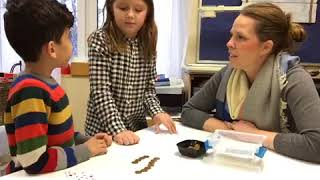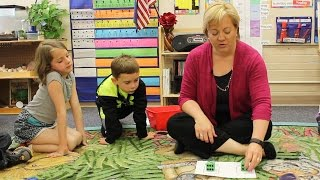Select Page

#### LessonsHelp teachers and students worldwide by sharing how you teach!
Create a lesson >>

>

## Adding & Subtracting (Operations & Algebraic Thinking)

Found 11 Results | Page 1 of 2## Finding the difference between two numbers

Jena Simms
##### Math

Adding & Subtracting (Operations & Algebraic Thinking)

Subtracting

##### Math

Adding & Subtracting (Operations & Algebraic Thinking)

##### Math

Adding & Subtracting (Operations & Algebraic Thinking)

Subtracting

##### Math

Adding & Subtracting (Operations & Algebraic Thinking)

##### Math

Adding & Subtracting (Operations & Algebraic Thinking)

##### Math

Adding & Subtracting (Operations & Algebraic Thinking)## Solving Addition Problems with Objects

Sumita Ghosh
##### Math

Adding & Subtracting (Operations & Algebraic Thinking)## Solving Subtraction Problems with Objects

Sumita Ghosh
##### Math

Adding & Subtracting (Operations & Algebraic Thinking)

Subtracting## Subtracting: Bigger and Smaller Number

Kathy Gray
##### Math

Adding & Subtracting (Operations & Algebraic Thinking)

Subtracting

A global movement of people sharing knowledge and learning from each other, to better educate our children and create hope for the world.

A global movement of people sharing knowledge and learning from each other, to better educate our children and create hope for the world.